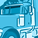# App Development

Announcements
Skip the ticket, Chat with Qlik Support instead for instant assistance.
cancel
Showing results for
Did you mean:Contributor II

## Aggr in dimension

Hi guys,

I am creating a buckets in bar chart and want to use it in dimension but unable to do that as in dimension we have to use aggr function.

Now the problem is I don't want to use aggr as I have to aggregarte it on some level.

I have multiple measures so can't aggregate on one level in dimension.

expression:

if(
(((sum({<Month={\$(vMaxMonth)}>}SalesNetValue)-sum({<Month={\$(VPrevMonth)}>}SalesNetValue))/sum({<Month={\$(VPrevMonth)}>}SalesNetValue))*100)>=0 and
(((sum({<Month={\$(vMaxMonth)}>}SalesNetValue)-sum({<Month={\$(VPrevMonth)}>}SalesNetValue))/sum({<Month={\$(VPrevMonth)}>}SalesNetValue))*100)<=5,'0-5 %',

if(
(((sum({<Month={\$(vMaxMonth)}>}SalesNetValue)-sum({<Month={\$(VPrevMonth)}>}SalesNetValue))/sum({<Month={\$(VPrevMonth)}>}SalesNetValue))*100)>5 and
(((sum({<Month={\$(vMaxMonth)}>}SalesNetValue)-sum({<Month={\$(VPrevMonth)}>}SalesNetValue))/sum({<Month={\$(VPrevMonth)}>}SalesNetValue))*100)<=10,'5-10 %',

if(
(((sum({<Month={\$(vMaxMonth)}>}SalesNetValue)-sum({<Month={\$(VPrevMonth)}>}SalesNetValue))/sum({<Month={\$(VPrevMonth)}>}SalesNetValue))*100)>10 and
(((sum({<Month={\$(vMaxMonth)}>}SalesNetValue)-sum({<Month={\$(VPrevMonth)}>}SalesNetValue))/sum({<Month={\$(VPrevMonth)}>}SalesNetValue))*100)<=25,'10-25 %'

)))

0 RepliesTags
Community Browser